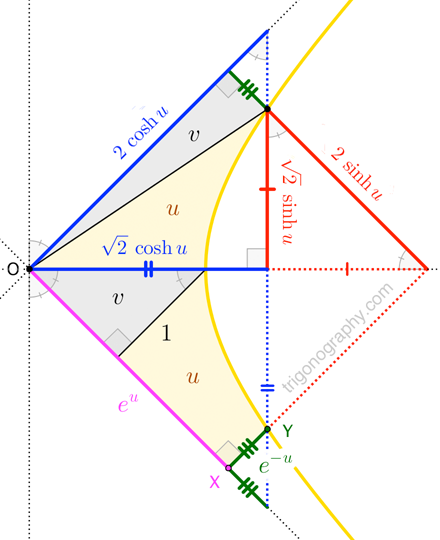## Exponential Forms of Hyperbolic Sine and Cosine\large \begin{align} 2\,\sinh u &\;=\; e^{u} -\, e^{-u} \\[4pt] 2\,\cosh u &\;=\; e^{u} + e^{-u} \end{align}
\begin{align} |\overline{OX}|\cdot|\overline{XY}| \;\equiv\; 1 &\quad\to\quad u = \int_{1}^{|\overline{OX}|} \frac{1}{t}dt \;=\; \ln |\overline{OX}| \\[6pt] &\quad\to\quad |\overline{OX}| = e^{u} \quad\text{and}\quad |\overline{XY}| = e^{-u} \end{align}Some background. Geometrically, we define $$\sinh u$$ and $$\cosh u$$ by direct analogy with $$\sin\theta$$ and $$\cos\theta$$: as certain perpendicular segments associated with an arc of
the “unit hyperbola”, $$x^2 – y^2 = 1$$.

While $$\theta$$ is usually interpreted as the length of a circular arc, we note that it is also twice the area of the corresponding circular sector. The hyperbolic parameter $$u$$ is interpreted via area, as well; today’s trigonograph shows why:

Conveniently scaling lengths in the unit hyperbola figure by $$\sqrt{2}$$ —and, thus, scaling areas by $$2$$— we see that $$\sinh u$$ and $$\cosh u$$ are directly computable from $$u$$ via the exponential function!
(In fact, we can say the same of $$\sin\theta$$ and $$\cos\theta$$, but we need complex exponentials for that.)

Taken from this answer of mine on the Mathematics Stack Exchange.